# Volume Converter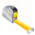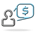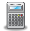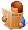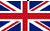Converter Currencies Calculator Articles

 Other units Area Energy Flowrate Force Length Mass Power Pressure Velocity Volume Temperature Time ______________ AcresAresBars Barrels per day Baryes BTUs BTUs per hour BTUs per minute BTUs per second Centimeters (cm) cm/sec Chains Cooking measures Crinals Cubic centimeters (cc) cc/sec cc-atmosphere Cubic decimeters Cubic feet Cubic feet per minute Cubic feet per second Cubic inches Cubic inches per minute Cubic inches per second Cubic meters Cubic meters per hour Cubic yards Cubic yards per minute Decimeters (dm) Drams DynesErgs per second Fluid drachmas Fluid ounces (fl.oz.) Feet (ft) Feet per hour Feet per minute Feet per second ft-lbf ft-lbf/hour ft-lbf/min ft-lbf/sec Furlongs Gallons Gallons per day Gallons per minute Gallons per second Gigawatts (GW) Gills Grains Grams (g) Gram-calories (g-cal) Hectares Hectometers Hundredweights (cwt) Inches (in) Inches H2O Inches Hg Inch-pound-forces (in-lbf) IST calories Joules (J) Joules per second (J/sec) Kilocalories (kcal) Kilograms (kg) kg-meters/sq. sec Kilogram-calories Kilogram-forces (kgf) Kgf/sq. cm Kgf/sq. meter Kilometers (km) Kilometers per hour (km/h) KiloPascal(kPa) Kilowatts (kW) Kilowatts per hour (kWh) Kips Knots Liters (l) Liters per minute Liters per second Long tons (imperial tons) MegaPascal(mPa) Megawatts (MW) Meters (m) Meters per minute Meters per second Metric horsepower Miles Miles per hour (mph) Miles per minute Milligrams (mg) Milliliters (ml) Millimeters (mm) Miner inches Newton (N) Ounces (oz) Ounce-force Pascal (Pa) Pints Pounds (lb) Pounds/sq. foot Pounds/sq. inch Poundals Poundals/sq. foot Poundals/sq. inch Pound-CHU Pound-force (lbf) Quarts Quarters Rods Shoe size Short tons/sq. footSlugs Square centimeters Square decimeters Square feet Square kilometers Square meters Square miles Square millimeters Square yards Stones Thermocalories TonsTons (refrigeration) Tonnes (metric tons) Torrs U.S. gallons U.S. horsepower per hour U.S. tons Watts Watts per hour Yards ________________ Ancient Greek: area Ancient Greek: currency Ancient Greek: length Ancient Greek: mass Ancient Greek: volume Ancient Roman: area Ancient Roman: length Ancient Roman: mass/coin Ancient Roman: volume Apothecary: imperial UK Apothecary: US/UK Apothecary: weightLeague units ________________ Calculator Contact Main website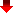Converting volume units is important in various applications, such as in cooking, pharmaceuticals, and chemical reactions. For instance, when cooking, it is essential to have accurate measurements of ingredients, and in many recipes, volume measurements are used. Therefore, it is crucial to know how to convert volume units, especially when converting between the metric and imperial systems.

In pharmaceuticals, the correct dosages of medicine depend on accurate volume measurements, and the use of proper conversion units is critical for ensuring patient safety. In chemical reactions, the precise measurement of the reactants is crucial for obtaining the desired outcome, and converting volume units helps in obtaining accurate measurements. For example, converting milliliters to fluid ounces, or liters to gallons, is important in ensuring the proper ratios of ingredients in a chemical reaction.

Converting volume units is necessary for a wide range of applications and helps in obtaining accurate measurements for various processes.

MetricToImperial.com